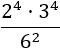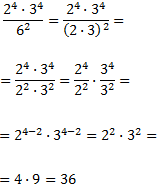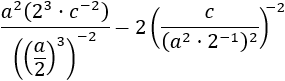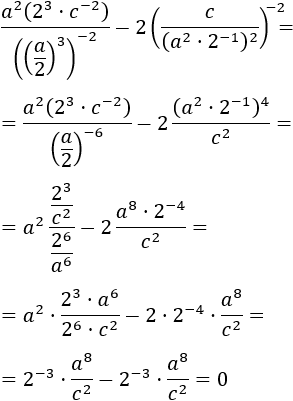# Properties of Exponents (Powers)

Author:
JLF
Topic:
Exponent

## 1. Introduction

Power is an expression of this type

that represents the result of multiplying the basea, by itself as many times as the exponent, b, indicates. We read it as "a to the power of b". Example 1: ﻿The base is 2 and the exponent is 3.

## 2. Exponents properties

Power of a product Product of powers (same base) Quotient of powers (same base) Power of a quotient Power of a power Negative exponent

## 3. Examples

Example 2:﻿ We have the multiplication of powers in the numerator, but we can't resolve it because there are different bases (2 and 3). In the denominator we have a power with a base of 6 (3·2). By writing the power in the denominator as the multiplication of powers of bases 3 and 2, we then have the same bases in the numerator and denominator and can now apply the rules.﻿ Example 3:﻿ The difficulty in this problem is the parameters, or what is the same, the letters. We work with them the same way as we do with numbers (the parameters represent numbers after all).